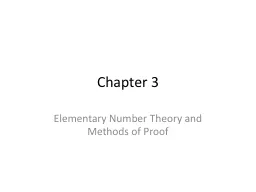# Chapter 3 Elementary Number Theory and Methods of ProofEmbed code:

## Chapter 3 Elementary Number Theory and Methods of Proof

Download Presentation - The PPT/PDF document "Chapter 3 Elementary Number Theory and M..." is the property of its rightful owner. Permission is granted to download and print the materials on this web site for personal, non-commercial use only, and to display it on your personal computer provided you do not modify the materials and that you retain all copyright notices contained in the materials. By downloading content from our website, you accept the terms of this agreement.

### Presentations text content in Chapter 3 Elementary Number Theory and Methods of Proof

Slide1

Chapter 3

Elementary Number Theory and Methods of Proof

Slide2

3.5

Direct Proof and Counterexample

5

Floor & Ceiling

Slide3

Floor & Ceiling

Definition

Floor

Given any real number

x

, the floor of x, denoted ⎣x⎦, is defined as: ⎣x⎦ = n ⇔ n ≤ x < n + 1.CeilingGiven any real number x, the ceiling of x, denoted ⎡x⎤, is defined as: ⎡x⎤ = n ⇔ n-1 < x ≤ n.

Slide4

Examples

Compute

x

⎦ and ⎡

x⎤ for the following:25/4 ⎣25/4⎦ = ⎣6+ 1/4⎦ = 6⎡25/4⎤ = ⎡6+ 1/4⎤ = 70.999⎣0.999⎦ = ⎣0 + 999/1000⎦ = 0⎡0.999⎤ = ⎡0 + 999/1000⎤ = 1

Slide5

Examples

The 1,370 soldiers at a military base a re given the opportunity to take buses into town for an evening out. Each bus holds a maximum of 40 passengers

What is the maximum number of buses the base will send if only full buses are sent?

⎣1,370/40⎦ = ⎣34.25⎦ = 34

How many buses will be needed if a partially full bus is allowed?⎡1,370/40⎤ = ⎡34.25⎤ = 35

Slide6

Does ⎣

x

+

y

⎦ = ⎣x⎦ + ⎣y⎦?Can you find a counterexample where the case is not true. If so, then you can prove that equality is false.How about x = ½ and y = ½ ?⎣½ + ½⎦ = ⎣1⎦ = 1⎣½⎦ + ⎣½⎦ = 0 + 0 = 0hence, the equality is false.

Slide7

Proving Floor Property

Prove that for all real numbers

x

and for all integers

m

, ⎣x + m⎦ = ⎣x⎦ + mSuppose x is a particular but arbitrarily chosen real number and m is particular but arbitrarily chosen integer.Show: ⎣x + m⎦ = ⎣x⎦ + mLet n = ⎣x⎦, n is integer n ≤

x < n+1n + m

x

+

m

<

n

+

m

m

to all sides)

x

+

m

⎦ =

n

+

m

(from previous)

since

n

= ⎣

x

Thus

x

+

m

⎦ = ⎣

x

⎦ +

m

Theorem 3.5.1

Slide8

Floor of n/2

Theorem 3.5.2 Floor of n/2

For any

n

, ⎣n/2⎦ = n/2 (if

n even) or (n-1)/2 (if n odd)ExamplesCompute floor of n/2 for the following:n = 5: ⎣5/2⎦ = ⎣2 ½⎦ = 2 = (5-1)/2 = 2n = 8: ⎣8/2⎦ = ⎣4⎦ = 4 = (8)/2 = 4

Slide9

Div / Mod and Floor

There is a relationship between div and mod and the floor function.

n

div

d

= ⎣n / d⎦ n mod d = n – d⎣n/d⎦From the quotient-remainder theorem, n = dq + r and 0≤r<d a relationship can be proven between quotient and floor.Theorem 3.5.3If n is any integer and d is a positive integer, and if q = ⎣n/d⎦ and r

= n – d⎣n/d⎦ then,

n

=

dq

+

r

and

0≤r<

d